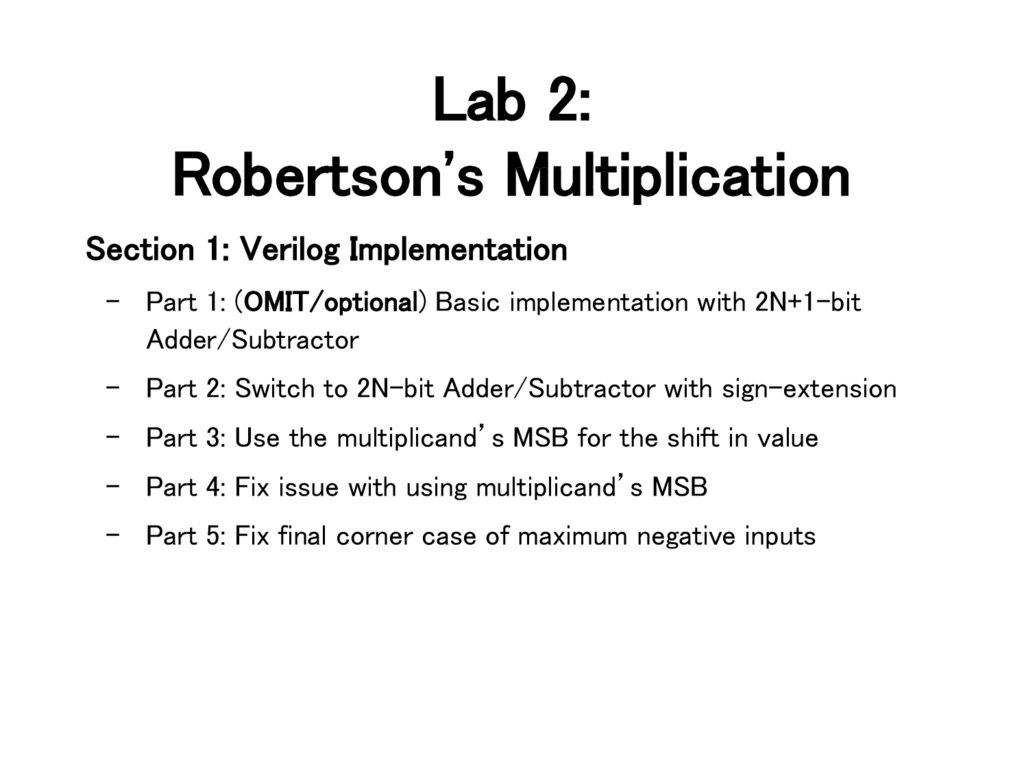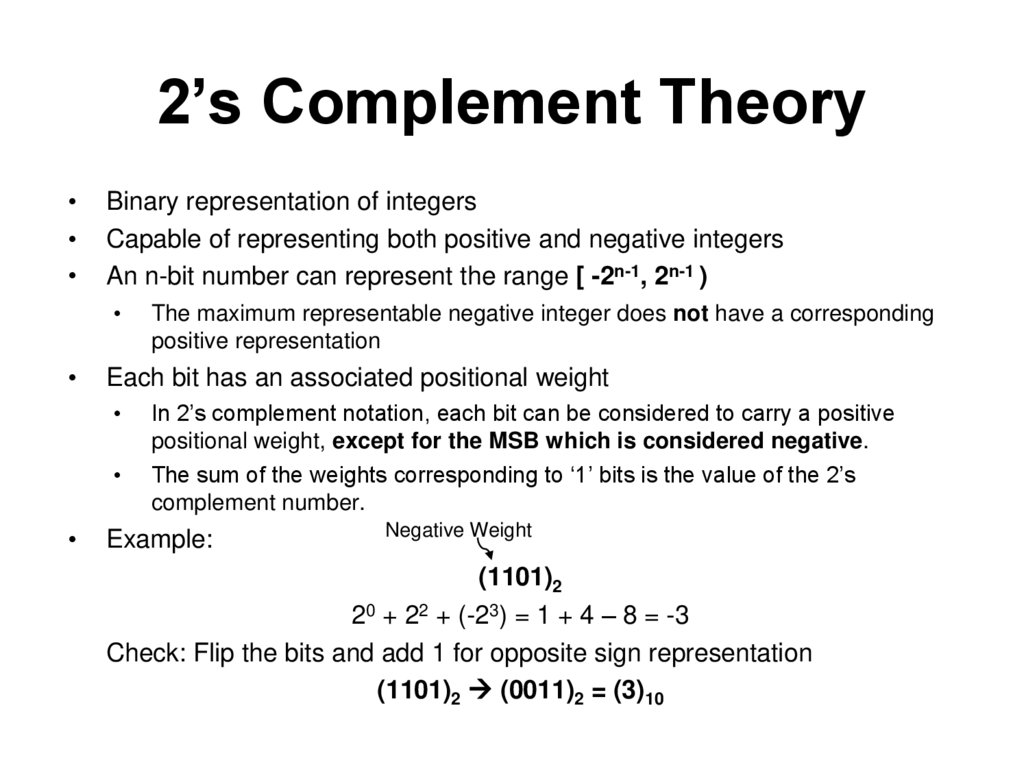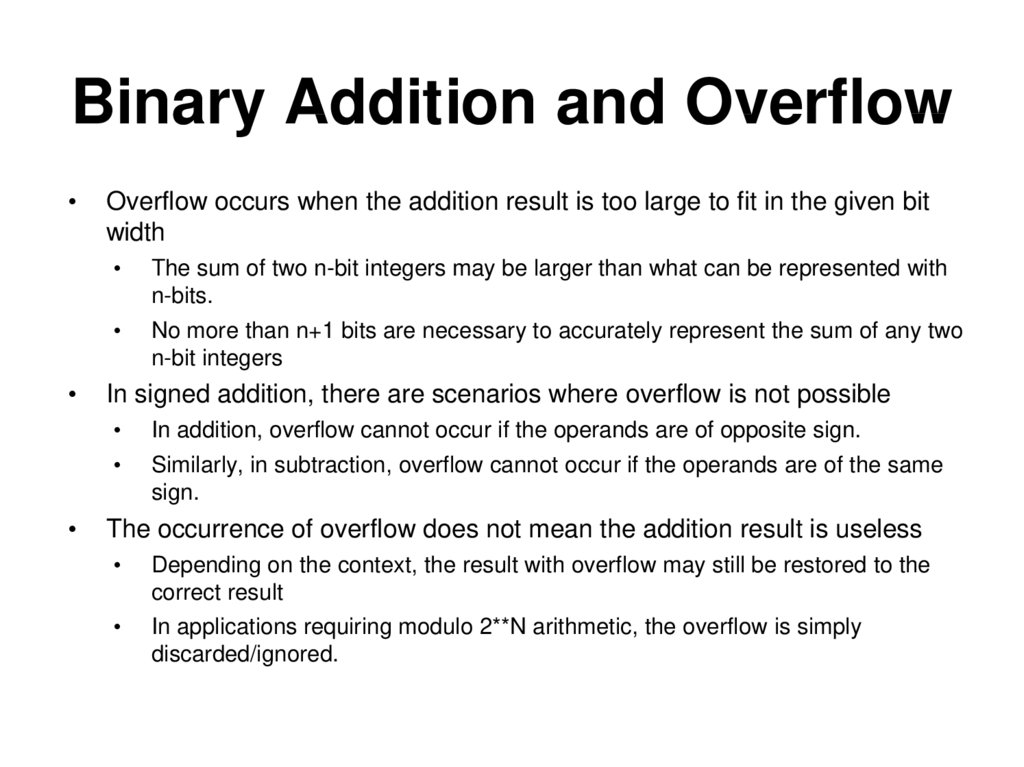# Robertson's multiplication

## 1.

Lab 2:
Robertson's Multiplication
Section 1: Verilog Implementation
– Part 1: (OMIT/optional) Basic implementation with 2N+1-bit
– Part 2: Switch to 2N-bit Adder/Subtractor with sign-extension
– Part 3: Use the multiplicand’s MSB for the shift in value
– Part 4: Fix issue with using multiplicand’s MSB
– Part 5: Fix final corner case of maximum negative inputs

## 2. 2’s Complement Theory

Binary representation of integers
Capable of representing both positive and negative integers
An n-bit number can represent the range [ -2n-1, 2n-1 )
The maximum representable negative integer does not have a corresponding
positive representation
Each bit has an associated positional weight
In 2’s complement notation, each bit can be considered to carry a positive
positional weight, except for the MSB which is considered negative.
The sum of the weights corresponding to ‘1’ bits is the value of the 2’s
complement number.
Example:
Negative Weight
(1101)2
20 + 22 + (-23) = 1 + 4 – 8 = -3
Check: Flip the bits and add 1 for opposite sign representation
(1101)2 (0011)2 = (3)10

## 3. Binary Addition and Overflow

Overflow occurs when the addition result is too large to fit in the given bit
width
The sum of two n-bit integers may be larger than what can be represented with
n-bits.
No more than n+1 bits are necessary to accurately represent the sum of any two
n-bit integers
In signed addition, there are scenarios where overflow is not possible
In addition, overflow cannot occur if the operands are of opposite sign.
Similarly, in subtraction, overflow cannot occur if the operands are of the same
sign.
The occurrence of overflow does not mean the addition result is useless
Depending on the context, the result with overflow may still be restored to the
correct result
In applications requiring modulo 2**N arithmetic, the overflow is simply

## 4. Overflow Examples

+
0110 (6)10
0101 (5)10
1011 (-5)10
+
0111 (7)10
1011 (-5)10
0010 (2)10
1101 (-3)10
+ 1010 (-6)10
1 0111 (-9)10
Overflow Possible
-
0101 (5)10
1101 (-3)10
1000 (-8)10
Overflow Not Possible
-
0110 (6)10
0101 (5)10
0001 (1)10
0101 (5)10
- 1101 (-3)10
0 1000 (8)10
Overflow Corrected
Extra headroom allows overflow to be corrected.
How do we determine the value of this bit?

## 5. How to Correct Overflow?

In this lab, you will explore several possibilities to develop a solution to fix
overflow in a multiplier circuit with n-bit inputs
Possible solutions include:
Using an n+1 bit adder to perform the addition during intermediary steps of the
multiplication algorithm.
Using an n-bit adder and sign extending the result during intermediary steps.
Straightforward implementation
Does not fix overflow, but perpetuates it
Matching the sign of the partial sums during intermediary steps with the
multiplicand’s sign.
Larger adder incurs area and delay penalties
Works (except for MSB of result), but introduces a bug for some cases that must
be fixed
Considering the signs of both the multiplier and multiplicand to determine the
sign of the final multiplication result.
Final solution

## 6. 2’s Complement Robertson’s Multiplication

Basic Idea
• Accumulate a partial sum in multiple steps.
• The rightmost bit of the multiplier is checked on every step to determine if
the multiplicand should be added to the partial sum.
• Shift the partial sum and the multiplier one bit to the right on every step.
Make the partial sum line up with the multiplicand;
Always check the rightmost bit of multiplier, no need to check higher bits;
Lower bits of the partial sum shifted into the multiplier register for storage.
Subtraction step at the end for negative multiplicands.

## 7. Multiplication Example

10102 * 11002
step1:
accumulator
multiplier
0000
1100
multiplicand
1010
right shift
1100
1010
00000000
0000000
111010
00110
00011000
multiplier
multiplicand
step2:
0000
0110
1010
right shift
step3:
(sub 1010)
result
correction step:
0000
0011
1010
accumulator = accumulator + multiplicand
1010
0011
1010
right shift
1101
0001
1010
accumulator = accumulator - multiplicand
0011
0001
1010
right shift
0001
Result: 00011000
1000
1010

## 8. Overall Guidelines: Glue Logic

• Use D Flip Flop En wo/SQ/ Provided
• The most significant bit of the accumulator during a shift
Different in each part of the lab
• The A/S signal for the adder/subtractor.
Add if A/S = 0; subtract if A/S = 1.

## 9. Implementation Summary

Acc. And
Width
Multiplicand
Register
Width
Accumulator Shift-in
(except last Shift)
Accumulator Shift-in
(last Shift only)
Part 1 17-Bit (OMIT)
16-Bit
Sign-extend Acc. MSB
Sign-extend Acc. MSB
Part 2 16-Bit
16-Bit
Sign-extend Acc. MSB
Sign-extend Acc. MSB
Part 3 16-Bit
16-Bit
MSB of multiplicand
Sign-extend Acc. MSB
Part 4 16-Bit
16-Bit
‘0’ until first ‘1’ in
multiplier, then MSB of
multiplicand
Sign-extend Acc. MSB
Part 5 16-Bit
16-Bit
‘0’ until first ‘1’ in
multiplier, then MSB of
multiplicand
Correct sign of
multiplication result

## 10. Verilog Components

Fill in the guts of:
N-bit counter-down
mux 2, 3, 5
N-bit and 2N-bit registers
Control unit FSM and microcircuits
Data path
robsmult: contains data path and control block
ROM – machine code instructions
shift_register – does logical or arithmetic right-shift
signed-mult – dummy block to test the testbench
toprobertson: contains robsmult (arguably excess layer)
upc_reg: program counter

## 11. Verilog Implementation

• Given Modules:
JAE_Lab2_assgn.zip: This is a full Robertson’s multiplier, but with the
details of the individual components left for you to fill in.
robertsontest.sv, the tesbench, is included in the .zip. You are
encouraged to insert additional operand value test cases – try a few
large and small numbers, some positive, some negative.
signed_mult.sv: This is a dummy behavioral multiplier to enable you to
test your testbench – do not use for your final turn in!
• Turn in:
See full list of turn-in components under TED/Content/Labs/Lab2